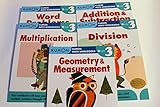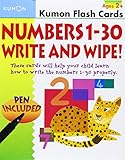### Numbers 1-30 Write & Wipe Flash Cards (Kumon Flash Cards)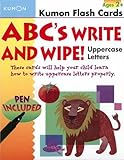### ABCs Uppercase Write & Wipe Flash Cards (Kumon Flash Cards)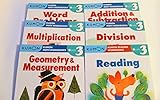### Kumon Grade 3 Set: Math Workbooks + Reading (6 Books)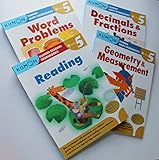### Kumon Grade 5 Math + Verbal workbooks (4 books) - Reading, Decimals & Fractions, Geometry & Measurement and Word Problem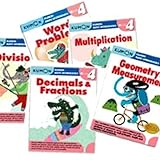### Kumon Grade 4 Math workbooks (5 books) - Decimals & Fractions, Multiplication, Division, Geometry & Measurement and Word Problem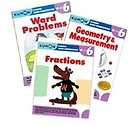### Kumon Grade 6 Math workbooks (3 books) - Fraction, Geometry & Measurement and Word Problem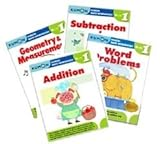### Kumon Grade 1 Math workbooks (4 books) - Addition, Subtraction, Geometry & Measurement and Word Problem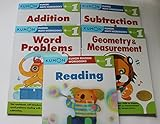### Kumon Grade 1 Math+Verbal (5 books) - Reading, Addition, Subtraction, Geometry & Measurement and Word Problem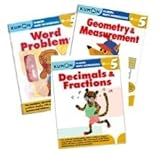### Kumon Grade 5 Math workbooks (3 books) - Fraction, Geometry & Measurement and Word Problem